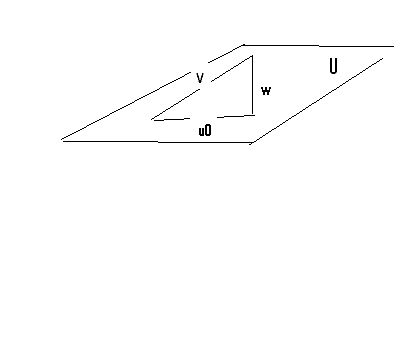# 8 orthogonal projection innequality

nhrock3
8)
$U=\{x=(x_{1},x_{2},x_{3},x_{4})\in R^{4}|x_{1}+x_{2}+x_{4}=0\}$
is a subspace of $R^{4}$
$v=(2,0,0,1)\in R^{4}$
find $u_{0}\in U$ so $||u_{0}-v||<||u-v||$
how i tried:
$U=sp\{(-1,1,0,0),(-1,0,0,1),(0,0,1,0)\}$
i know that the only $u_{0}$ for which this innequality will work
is if it will be the orthogonal projection on U paralel to v
i am not sure about the theory of finding it
what to do next?

Last edited by a moderator:

Mentor
8)
$$U=\{x=(x_{1},x_{2},x_{3},x_{4})\in R^{4}|x_{1}+x_{2}+x_{4}=0\}$$
is a subspace of $R^{4}$
$v=(2,0,0,1)\in R^{4}$
find $u_{0}\in U$ so $||u_{0}-v||<||u-v||$
how i tried:
$U=sp\{(-1,1,0,0),(-1,0,0,1),(0,0,1,0)\}$
OK, this is a basis for U.
i know that the only $u_{0}$ for which this innequality will work
is if it will be the orthogonal projection on U paralel to v
i am not sure about the theory of finding it
what to do next?
You can think of U as being a plane through the origin in R4. It doesn't hurt to visualize this as a plane in three dimensions. U is actually a hyperplane, being a space of one dimension less than the space it's in, but it's more convenient to think of this problem as a plane in R3.

Vector v is not in the "plane" (U), so the vector u0 that is closest to v will be the vector that is directly under v, and lying in U. In other words, u0 is the projection of v onto the "plane" U. Surely in what you're studying there are some examples of how to find the projection of one vector onto another vector or a vector onto a plane.

nhrock3
actually there no examples
:)

MentorThe triangle is a right triangle, so u0 $\cdot$ w = 0.